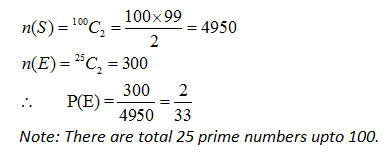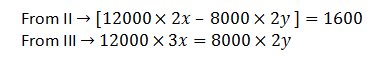# IAS Exam: IAS Prelims CSAT General Mental Ability Set 4

The CSAT Paper of IAS Prelims Exam has been qualifying in nature from last year but in case, if a candidate could not be able to score the required qualifying marks in CSAT Paper then his/her other papers will not be evaluated. So, it is important for the candidates to do preparation of IAS Prelims Exam in such a manner that there must not put them in any suspicion of underscoring. Here, we have provided the questions of General Mental Ability for the Civil Services CSAT Paper II of UPSC IAS Exam 2016.

Apr 29, 2016 17:35 IST

In the Civil Services Prelim Examination, the CSAT paper has been made qualifying in nature from 2015 onwards. The candidates have to score at least qualifying marks in the paper but in case, if a candidate could not be able to score the required qualifying marks in CSAT Paper then his/her other papers will not be evaluated. So, it is important for the candidates to do preparation in such a manner that there must not lay them in any suspicion of underscoring.

Here, we have provided the questions of General Mental Ability for the Civil Services CSAT Paper II of UPSC IAS Exam 2016. The pattern of these General Mental Ability Multiple Choice Questions has been kept in line with the questions asked in earlier attempts of Civil Services IAS Exam.

Probability

1. In a lucky draw of 100 tokens numbered 1 to 100, two tokens are drawn simultaneously. Find the probability that both the tokens drawn have prime numbers.Explanation:Explanation:a) 56

b) 23

c) 13

d) 17

Explanation:Now, since 13 is not the factor of either 391 or 392.

Hence, option c) is the correct answer.

Mensuration

4. From a solid sphere of radius 25 cm, a right circular cylindrical hole of radius 15 cm whose axis passing through the centre is removed. The total surface area of the remaining solid is:Explanation: The exact value of the required surface area cannot be found using the general formula. For the exact value of total surface area we have to solve it with the help of calculus. In the following solution we are calculating lump sum value of total surface area.

Now,Data Interpretation

Directions (5 - 8) – The bar diagram given below represents the annual percentage growth of sale of Television sets over the years. Study the bar diagram carefully and answer the questions based on it:5. If the difference between the sale of LCD TV sets in 2013 and 2014 was 4500, then what was the number of LCD TV sold in 2014?

a) 54000

b) 18000

c) 29500

d) 19500

Explanation : Percentage growth in the sale of LCD TV sets over 2013 to 2014 is 18%.

When the difference is 18, the number of LCD TV sold in 2014 is 118.

Therefore, when the difference is 4500, the number of LCD TV sold in6. In which of the following years was there a minimum percentage growth in sales of LED TV?

a) 2012

b) 2013

c) 2014

d) 2015

Explanation: The minimum percentage growth in sales of LED TV is 15% and it is in 2012.

7. Approximately, what was the average of percentage growth in sale of LCD TVs over the given years?

a) 16

b) 18

c) 22

d) 208. If the total number of LED TV sold in 2014 was 140000, then what was the number of LED TVs sold in 2015?

a) 160000

b) 168000

c) 170000

Explanation:

Percentage growth in 2015 = 20%Data Sufficiency

Directions (9 -10): In each of the questions below consists of a question and two statements numbered I and II given below it. You have to decide whether the data provided in the statements are sufficient to answer the question. Read both the statements and Give answer:

9. The rate of simple interest at which Ramit has taken money from Madan is:

I.  Madan gives Rs. 20000 to Ramit and Sumit in the ratio 3:2.
II. The difference of the interest returned by them after 2 yrs is Rs. 1600.
III The interest paid by Ramit after 3 yrs is equal to interest paid by Sumit after 2 yrs.

a) I alone is sufficient while II alone is not sufficient.

b) II alone is sufficient while I alone is not sufficient.

c) I, II and III together are sufficient.

d) Both I and II are sufficient.

Explanation: Let x and y be the rate of interest of Ramit and Sumit of one year.
From I →Ramit‘s & Sumit‘s money are Rs. 12000 and Rs. 8000 respectively.Hence, all the three statement are required to solve the question.

10. The height of a right angled triangle is?

I. 16 cm is the perimeter of a rectangle.
II. 12 square cm is the area of a triangle.
III. The breadth of a rectangle is equal to the base of the triangle and the area of the triangle is equal to area of rectangle.

a) Only I
b) Only II
c) Any two of them
d) I, II and III together